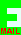## PRIZE PUZZLE FOR JANUARY 2004

Whether or not there is a Hot Key magazine, this is the puzzle for January 2004.

#### Balancing the Equation

Using the decimal digits 1 to 9 once and once only, construct an equation that uses only the plus sign (as many times as needed). There are many solutions so they should be quite easy to discover with a little testing and a pencil and paper. Here are some examples that are wrong but show the kind of answer expected:

19 + 76 = 34 + 52 + 8
Wrong because the equation does not balance.

1089 = 765 + 324
Wrong because there is a zero.

63 + 15 = 34 + 28 + 7 + 9
Wrong because the digit 3 is present twice.

If you can find a solution that uses only one plus sign you will get a special mention and will be included in the draw twice.

Send your solution to me, David Broughton, by e-mail or snail mail to arrive by Wednesday 4th February 2004. The e-mail link is.

[home] [back]